### IDENTIFY Statement

`IDENTIFY` options ;

The IDENTIFY statement produces plots of the sample autocorrelation function (ACF) and partial autocorrelation function (PACF) for identifying the ARIMA part of a regARIMA model. The sample ACF and PACF are produced for all combinations of the nonseasonal and seasonal differences of the data specified by the DIFF= and SDIFF= options.

The original series is first transformed as specified in the TRANSFORM statement.

If the model includes a regression component (specified using the REGRESSION, INPUT, and EVENT statements or the MDLINFOIN= data set in the PROC X12 statement), both the transformed series and the regressors are differenced at the highest order that is specified in the DIFF= and SDIFF= option. The parameter estimates are calculated using the differenced data. Then the undifferenced regression effects (with the exception of a constant term) are removed from the undifferenced data to produce undifferenced regression residuals. The ACFs and PACFs are calculated for the specified differences of the undifferenced regression residuals.

If the model does not include a regression component, then the ACFs and PACFs are calculated for the specified differences of the transformed data.

Tables displayed in association with identification are Autocorrelation of Model Residuals and Partial Autocorrelation of Model Residuals. If the model includes a regression component (specified using the REGRESSION, INPUT, and EVENT statements or the MDLINFOIN= data set in the PROC X12 statement), then the Regression Model Parameter Estimates table is also displayed if the PRINTREG option is specified.

The following options can appear in the IDENTIFY statement:

DIFF=(order, order, order)

specifies orders of nonseasonal differencing to use in model identification. The value 0 specifies no differencing, the value 1 specifies one nonseasonal difference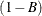, the value 2 specifies two nonseasonal differences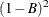, and so forth. The ACFs and PACFs are produced for all orders of nonseasonal differencing specified, in combination with all orders of seasonal differencing that are specified in the SDIFF= option. The default is DIFF=(0). You can specify up to three values for nonseasonal differences.

MAXLAG=value

specifies the number of lags for the sample autocorrelation function (ACF) and partial autocorrelation function (PACF) of the regression residuals for model identification. The default is 36 for monthly series and 12 for quarterly series. MAXLAG applies to both tables and plots. The minimum value for MAXLAG= is 1.

PRINTREG

causes the Regression Model Parameter Estimates table to be printed if the REGRESSION statement is present. By default, this table is not printed.

SDIFF=(order, order, order)

specifies orders of seasonal differencing to use in model identification. The value 0 specifies no seasonal differencing, the value 1 specifies one seasonal difference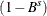, the value 2 specifies two seasonal differences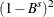, and so forth. The value for s corresponds to the period specified in the SEASONS= option in the PROC X12 statement. The value of the SEASONS= option is supplied explicitly or is implicitly supplied through the INTERVAL= option or the values of the DATE= variable. The ACFs and PACFs are produced for all orders of seasonal differencing specified, in combination with all orders of nonseasonal differencing specified in the DIFF= option. The default is SDIFF=(0). You can specify up to three values for seasonal differences.

For example, the following statement produces ACFs and PACFs for two levels of differencing:and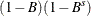:

```   identify diff=(1) sdiff=(0, 1);
```